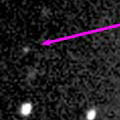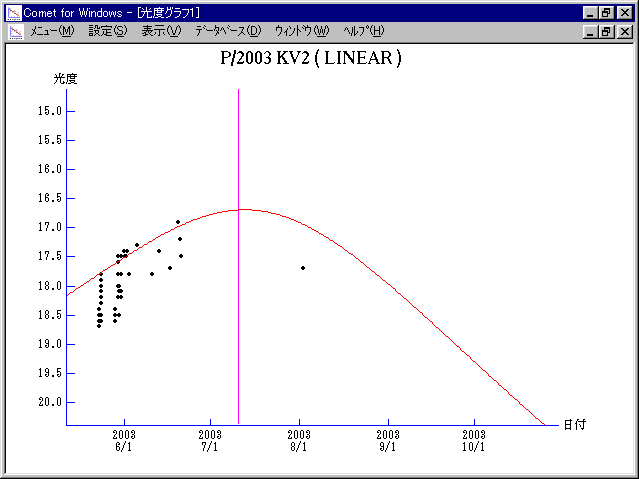# \$B%j%K%"WB@1(B

197P/LINEAR (2003)###\$B%W%m%U%#!<%k(B

 \$BId9f(B 197P/2003 KV2 \$BH/8+F|(B 2003\$BG/(B5\$B7n(B23\$BF|(B \$BH/8+8wEY(B 18.4\$BEy(B \$BH/8+ Lincoln Laboratory Near-Earth Asteroid Research project

###\$B###\$B50F;MWAG(B

```Epoch 2003 July 20.0 TT = JDT 2452840.5
T 2003 July 10.84758 TT                                 Kobayashi
q   1.0630199            (2000.0)            P               Q
n   0.20290915     Peri.  188.74167     -0.26990879     +0.87808941
a   2.8681485      Node    66.40774     -0.85531838     -0.03017958
e   0.6293707      Incl.   25.54003     -0.44224396     -0.47754390
P   4.86
From 304 observations 2003 May 23-2013 Sept. 25, mean residual 0".7.
Nongravitational parameters A1 = +0.00, A2 = +0.0018.
```

###\$B@1?^(B###\$B8wEYJQ2=(B

```        m1 = 17.5 + 5 log\$B&\$(B + 10.0 log r
```##### \$B50F;MWAG\$O(BM.P.E.C. 2015-M77\$B\$K7G:\\$5\$l\$?\$b\$N\$G\$9!#(B \$B@1?^\$O(B StellaNavigator Ver.2.0 for Windows (\$B%"%9%H%m%"!<%D(B \$BJTCx(B / \$B%"%9%-!<=PHG6I4)(B) \$B\$G:n@.\$7\$?\$b\$N\$G\$9!#(B \$B8wEY%0%i%U\$O(BComet for Windows\$B\$G:n@.\$7\$?\$b\$N\$G\$9!#(B